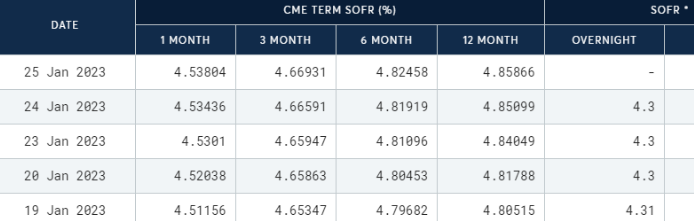I want to better understand the difference between the 1-month and 3-month SOFR rate, and when to use each for cash flow discounting.

For example - I have a 5 year project, and someone has decided to use the 3-month SOFR rate. My question is why? What does this assume about the movement of cash flows?

Furthermore, I would like to know if there's a mathematical relationship between the two (1-month vs 3-month).

Some Googling led to the following definition

Three-Month Term SOFR means the rate for Term SOFR for a tenor of three months that is published by the Term SOFR Administrator at the Reference Time for any interest period, as determined by the Calculation Agent after giving effect to the Three-Month Term SOFR Conventions.

Does this definition provide any leads? Can I define the 3-month SOFR as F(t,T) to be the forward rate of the 3-month SOFR at some forward time t?, and T=3 is fixed?

Are the forward curves then shown in the columns below?Thank you very much